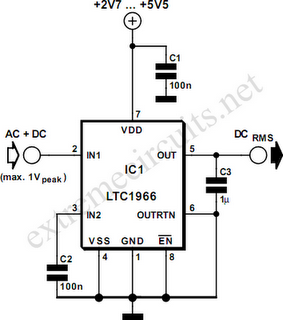# Learning Electronics

Learn to build electronic circuits

# RMS to DC Converter

In order to measure the RMS value of an alternating voltage an accurate converter is required to produce the true RMS value of its alternating input as a DC output. With simple sinewave inputs the RMS voltage can simply be calculated as 0.707 times the peak AC voltage, but with complex waveforms the calculation is not nearly as straightforward. The RMS value is defined as the DC voltage that would give the same heating effect in a resistor as the alternating voltage. The LTC 1966 from Linear Technology (www.linear-tech.com) uses a new form of delta-sigma conversion and is designed for battery operation, drawing only 170µA from the supply. The new technique is accurate to 0.02 % between 50 mV and 350 mV and is highly linear. It can operate from 50 Hz to 1 kHz (with an error of 0.25 %) and up to 6 kHz with a 1 % error.The input voltage range on the differential inputs IN1 and IN2 extends to the supply rails, and so in the non-symmetrical circuit shown here the voltage on IN1 can swing between 0 V and the supply voltage. If the signal to be measured is AC only, then another coupling capacitor will be required. The input impedance is many megohms. The output voltage at the OUT pin can be offset by applying a DC voltage to the OUTRTN pin. This is particularly helpful when using the device with LCD multimeter ICs such as the 7106. A further capacitor is connected to the output which is charged up to the required voltage by the switched-capacitor circuit in the converter. The capacitor required is ten times smaller than that demanded by previous RMS to DC converter designs. The LTC1966 is not temperature sensitive and is available in an 8-pin MSOP package. It allows a tiny RMS to DC converter to be constructed using just four components.